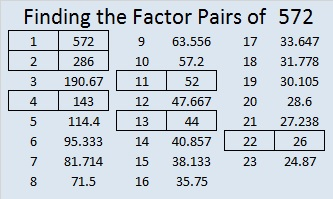# 572 and Level 1

72 can be evenly divided by 4 so 572 is divisible by 4.

5 – 7 + 2 = 0, which can be evenly divided by 11, so 11 is a factor of 572.

572 is the hypotenuse of the Pythagorean triple 220-528-572. Which factor of 572 is the greatest common factor of those three numbers?Print the puzzles or type the solution on this excel file: 10 Factors 2015-08-03

—————————————————————————————————

• 572 is a composite number.
• Prime factorization: 572 = 2 x 2 x 11 x 13, which can be written 572 = (2^2) x 11 x 13
• The exponents in the prime factorization are 2, 1, and 1. Adding one to each and multiplying we get (2 + 1)(1 + 1)(1 + 1) = 3 x 2 x 2 = 12. Therefore 572 has exactly 12 factors.
• Factors of 572: 1, 2, 4, 11, 13, 22, 26, 44, 52, 143, 286, 572
• Factor pairs: 572 = 1 x 572, 2 x 286, 4 x 143, 11 x 52, 13 x 44, or 22 x 26
• Taking the factor pair with the largest square number factor, we get √572 = (√4)(√143) = 2√143 ≈ 23.91652—————————————————————————————————